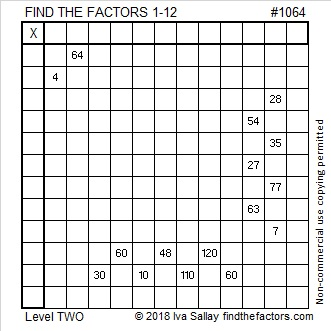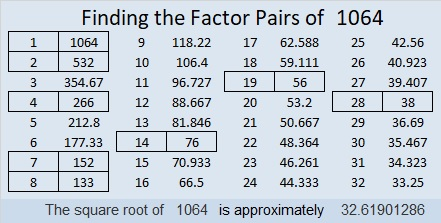# 1064 and Level 2

If you did yesterday’s puzzle, then you will recognize four of the clues in today’s puzzle. They will give you a good start in finding all the rest of factors. See how well you do on this one!Print the puzzles or type the solution in this excel file: 12 factors 1063-1072

Now I’ll tell you a little bit about the number 1064:

Its 0 is an even digit, and its last two digits, 64, can be evenly divided by 8, so 1064 is also divisible by 8.

• 1064 is a composite number.
• Prime factorization: 1064 = 2 × 2 × 2 × 7 × 19, which can be written 1064 = 2³ × 7 × 19
• The exponents in the prime factorization are 3, 1, and 1. Adding one to each and multiplying we get (3 + 1)(1 + 1)(1 + 1) = 4 × 2 × 2 = 16. Therefore 1064 has exactly 16 factors.
• Factors of 1064: 1, 2, 4, 7, 8, 14, 19, 28, 38, 56, 76, 133, 152, 266, 532, 1064
• Factor pairs: 1064 = 1 × 1064, 2 × 532, 4 × 266, 7 × 152, 8 × 133, 14 × 76, 19 × 56, or 28 × 38
• Taking the factor pair with the largest square number factor, we get √1064 = (√4)(√266) = 2√266 ≈ 32.61901The difference in the numbers in one of its factor pairs, 28 × 38, is exactly ten, so we are exactly 25 away from the next perfect square number:
33² – 5² = 1089 – 25 = 1064

I like the way 1064 looks in a couple of other bases:
It’s 888 in BASE 11 because 8(11² + 11 + 1) = 8(133) = 1064, and
it’s 248 in BASE 22 because 2(22²) + 4(22) + 8(1) = 1064

This site uses Akismet to reduce spam. Learn how your comment data is processed.